# 5 graph problems you should know in Java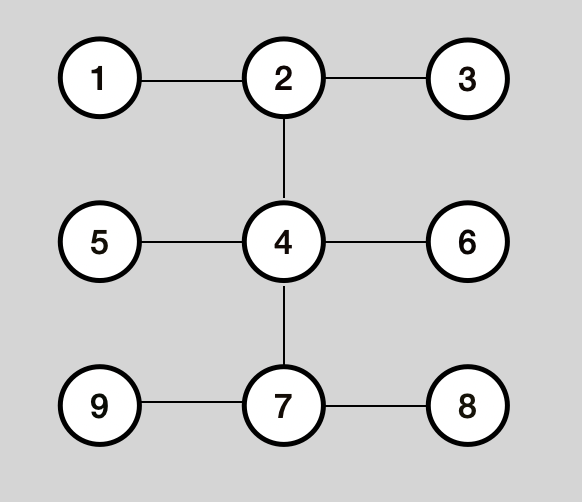A `Graph` is a collection of nodes and edges. Graphs are really useful to explain relationships between things, for instance we can represent a map of cities with a graph.

We have directed and undirected graphs. The first type of graphs in order to navigate from node A to node B there must be an edge with a direction from A to B. In undirected graphs if there’s an edge between A and B we can navigate from A to B and from B to A.

## How can we implement graph data structure

There are 2 ways of representing a Graph, one is with a `adjacency matrix` and another way is with an `adjacency list`. The second one is normaly the way to go and is the most common way to represent a `Graph`.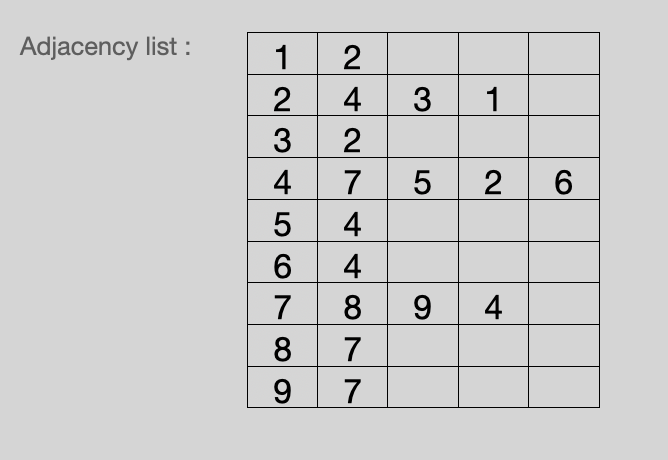In Java it can be implemented with a `Map` with keys as nodes and as value the list of nodes that are adjacent to that key. Another way to represent a graph is with `LinkedList` where each value of this data structure is also a `LinkedList` with all the nodes adjacent to that node in that position. Even that a node has no neighbours it should appear in the map but with empty value.

## Breath first search (BFS)

In this algorithm we traverse the `Graph` using a `Queue`. In the course of the traversal every node of the Graph changes it’s state from `unvisited` to `visited`. Only when we have visited all the adjacent nodes from the current node we move to the next node.

``````
public class Graph {

private final int V;
private final Queue<Integer> queue;

public Graph(int v){
this.V = v;
for (int i=0; i<v; i++){
}
}
}

public void BFS(int n){
boolean visited[] = new boolean[V];
visited[n]=true;
while (queue.size() != 0){
n = queue.poll();
System.out.print(n + " ");
if (!visited[a]){
visited[a] = true;
}
}
}
}

}

``````

## Depth first search (DFS)

In this case we start in a node and we pick a direction and continue in the same direction until we cannot continue. You are exploring one direction as far as you can before switching directions. This algorithm uses an `stack` in his iterative version. We have to see as a vertical data structure.

``````public void DFS(int n){
boolean visited[] = new boolean[V];
Stack<Integer> stack = new Stack<>();
stack.push(n);
while(!stack.empty()) {
n = stack.peek();
stack.pop();
if(visited[n] == false) {
System.out.print(n + " ");
visited[n] = true;
}
if (!visited[a]){
stack.push(a);
}
}
}
}

``````

## Problem 1 - Find if there’s a path between 2 nodes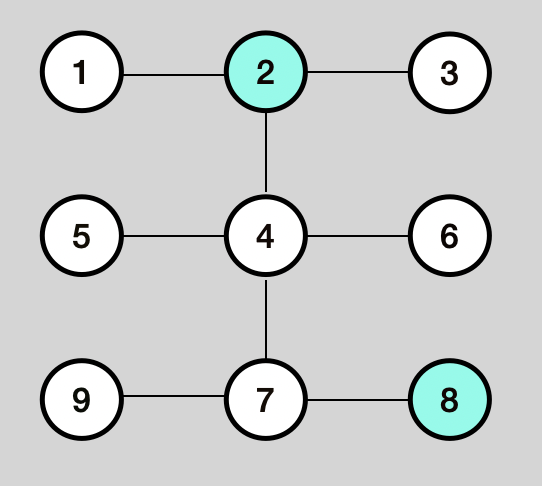`````` public boolean hasPath(int src, int dest, boolean []visited){
if(visited[node]){
return false;
}
if(src==dst) return true;
visited[node]=true;
if(hasPath(neighbor, dst, visited)){
return true;
}
}
return false;
}

``````

## Problem 2 - Count the number of connected components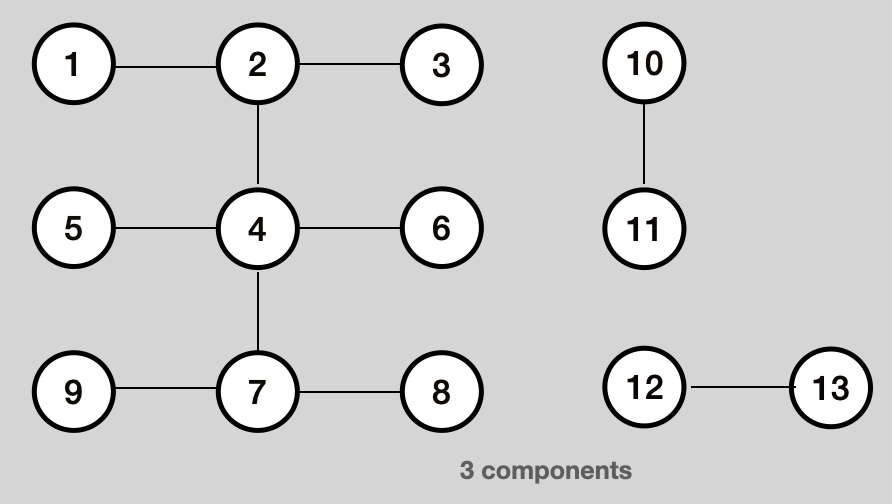``````public int connectedComponentsCount(){

boolean visited[] = new boolean[V];
int count = 0;
for (int i = 0; i < V; i++){
if (explore(i, visited)){
count++;
}
}
return count;
}

public boolean explore(int node, boolean visited[]){

if(visited[node]){
return false;
}
visited[node]=true;
explore(graph, neighbor, visited);
}
return true;
}
``````

## Problem 3 - Find the largest component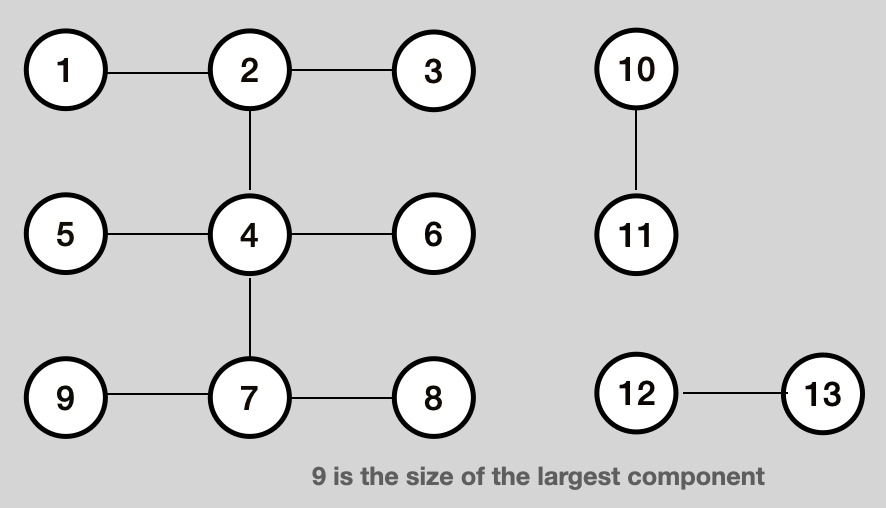``````public int largestComponent() {
boolean []visited = new boolean[V];
int largest = 0;
for (int i = 0; i < V; i++) {
int result = exploreSize(i, visited);
if (result > largest) {
largest = result;
}
}
return largest;
}

public int exploreSize(int node, boolean[] visited) {
if (visited[node]) {
return 0;
}
visited[node] = true;
int size = 1;
for (int neighbor : adj[node]) {
size += exploreSize(neighbor, visited);
}
return size;
}
``````

## Problem 4 - Find the shortest path between 2 nodes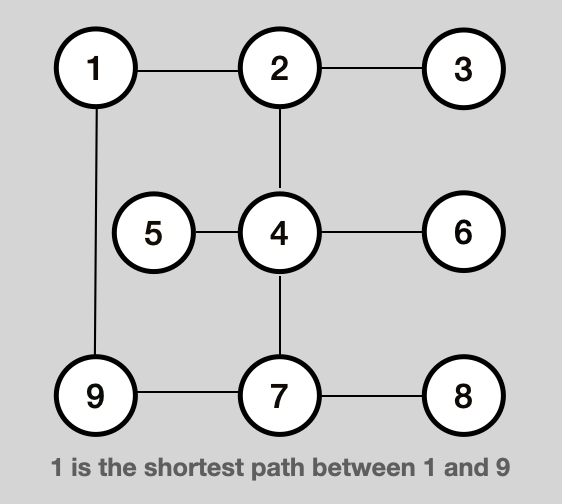``````
class Node{
int value;
int distance;
}

Queue<Node> queue = new ArrayDeque<>();

public int shortestPath(int src, int dest) {
boolean[] visited = new boolean[V];
visited[src] = true;
while (queue.size() != 0) {
Node node = queue.poll();
if (node.value == dest) {
return node.distance;
}
System.out.print(node.value + " ");
for (int a : adj[node.value]) {
if (!visited[a]) {
visited[a] = true;
}
}
}
return -1;
}

public static void main(String args[]) {
Graph g = new Graph(7);

System.out.println("Shortest path: " + g.shortestPath(4, 0));
}

``````

## Problem 5 - Count the number of islands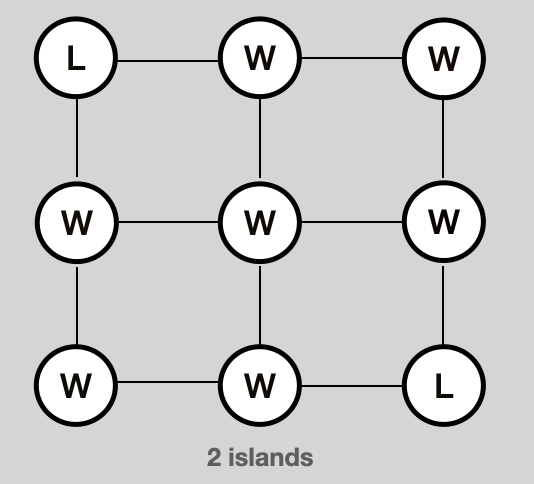``````
public class IslandCounter {

public int countIslands(char[][] grid) {

boolean[][] visited = new boolean[grid.length][grid.length];
int islands = 0;
for (int row = 0; row < grid.length; row++) {
for (int column = 0; column < grid.length; column++) {
if (explore(grid, row, column, visited)) {
islands++;
}
}
}
return islands;
}

public boolean explore(char[][] grid, int row, int column, boolean[][] visited) {

boolean rowInbounds = 0 <= row && row < grid.length;
boolean columnInbounds = 0 <= column && column < grid.length;

if (!rowInbounds || !columnInbounds) return false;

if (visited[row][column]) return false;

if (grid[row][column] == 'W') return false;

visited[row][column] = true;

explore(grid, row - 1, column, visited);
explore(grid, row + 1, column, visited);
explore(grid, row, column - 1, visited);
explore(grid, row, column + 1, visited);

return true;
}

public static void main(String[] args) {

IslandCounter islandCounter = new IslandCounter();

char [][] grid = {   {'L','L', 'W','W', 'L'}
,{'L','L', 'W','W', 'W'}
,{'W','W', 'W','W', 'W'}
,{'W','W', 'W','L', 'L'}
,{'L','W', 'W','L', 'L'}};

System.out.println("Number of islands in this grid :" +islandCounter.countIslands(grid));
}
}

``````

### Conclusion

In this post we have seen how to define a graph data structure in Java. We have seen 5 problems related with graphs and how to implement them using Java. We have seen 2 key algorithms that help us solve this kind of problems, DFS and BFS.

I won't give your address to anyone else, won't send you any spam, and you can unsubscribe at any time.
Disclaimer: Opinions are my own and not the views of my employer

Updated: